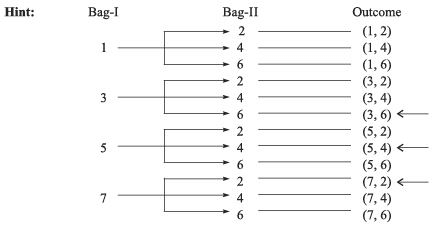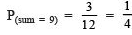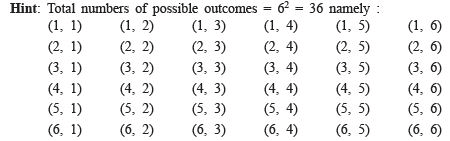# HOTS Questions: Probability Notes | Study Mathematics (Maths) Class 9 - Class 9

## Class 9: HOTS Questions: Probability Notes | Study Mathematics (Maths) Class 9 - Class 9

The document HOTS Questions: Probability Notes | Study Mathematics (Maths) Class 9 - Class 9 is a part of the Class 9 Course Mathematics (Maths) Class 9.
All you need of Class 9 at this link: Class 9

1. A bag-I contains four cards numbered 1, 3, 5 and 7 respectively. Another bag-II contains three cards numbered 2, 4 and 6 respectively. A card is drawn at random from each bag. Find the probability that the sum of two cards drawn is 9.All outcomes = 12Favourable outcomes = 3⇒2. A bag contains 7 white, 3 red and 4 black balls. A ball is drawn at random. Find the probability that it is a red or a black ball.

 Hint: Total number of possible outcomes = 7 + 3 + 4 = 14. Out of 14 possible outcomes, there are 7 favourable outcomes (3 red and 4 black) i.e. there are 7 balls that are either red or black.∴ Required probability = P(a red or a black ball) = (7/14)= (1/2)

3. In a single throw of two dice, find the probability of getting a total of 8.∴ Favourable outcomes are : (2, 6), (3, 5), (4, 4), (5, 3), (6, 2) i.e. 5 outcomes are favourable.∴ Required probability = (5/36)

4. One card is drawn from a well-shuffled deck of 52 cards. Find the probability of getting a face card.

 Hint: 52 cards are divided into 4 suits of 13 cards each. Kings, queen and jacks are called face-cards.∴ Total number of face cards = 3 × 4 = 12∴ Favourable outcomes = 12⇒ Required probability = (12/52)= (3/13)

5. Two unbiased coins are tossed once. What is the probability of getting exactly one head?

 Hint: In a throw of two coins, the total number of possible outcomes = 22 = 4 [namely : (H, H), (H, T), (T, H) and (T, T)].For the event of getting exactly one head (i.e. no tail).⇒ Favourable outcomes are (H, T) and (T, H) i.e., 2⇒ Required probability = (2/4)= (1/2)
The document HOTS Questions: Probability Notes | Study Mathematics (Maths) Class 9 - Class 9 is a part of the Class 9 Course Mathematics (Maths) Class 9.
All you need of Class 9 at this link: Class 9Use Code STAYHOME200 and get INR 200 additional OFF

## Mathematics (Maths) Class 9

73 videos|352 docs|109 tests

Track your progress, build streaks, highlight & save important lessons and more!

,

,

,

,

,

,

,

,

,

,

,

,

,

,

,

,

,

,

,

,

,

;